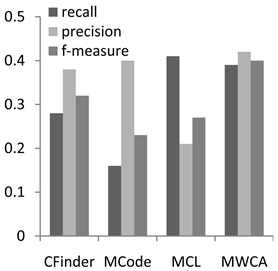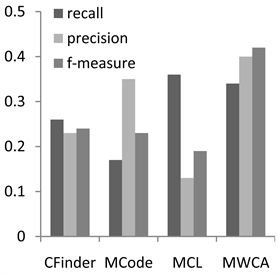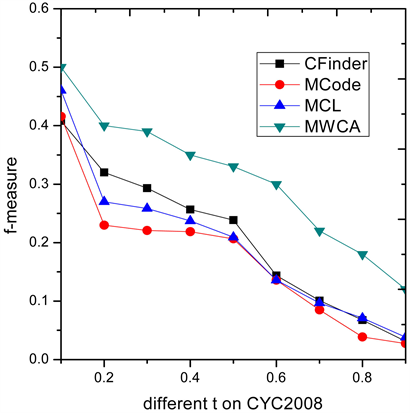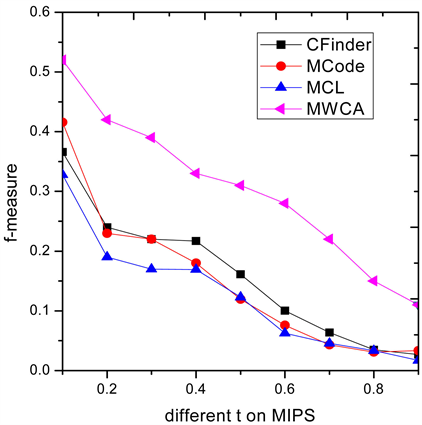# 一种新的基于多源信息的复合物识别算法A New Method for Protein Complex Detection Based on Multi-Source Information

• 全文下载: PDF(1026KB)    PP.67-75   DOI: 10.12677/BIPHY.2018.6464007
• 下载量: 354  浏览量: 801   科研立项经费支持

Protein complexes are important biological function modules in protein interaction (PPI) network and play an important role in biological activities. Usually the topological structure is used as the condition for clustering in the PPI network, which constrains the overall performance of complex identification. To improve this problem, a new complex identification algorithm is proposed based on fusion of multi-source information. Firstly, based on the semantic similarity of GO, we construct a weighted PPI model and design a core-attachment clustering algorithm based on the density, diameter and cosine similarity. Secondly, the seed nodes are sequentially selected according to the descending order of the node degrees in the network and expanded in weighted graph model to identify protein complex. Lastly, the proposed method is compared with three classical algorithms. The experimental results indicate that the algorithm can effectively enhance the performance in terms of precision, F-measure and functional enrichment analysis.

1. 引言

2. 基于多源信息的复合物识别算法(MWCA)

2.1. 融合基因本体的有权网络模型

$si{m}_{MAX}\left(\text{X},\text{Y}\right)=MA{X}_{{l}_{1}\in GO\left(\text{X}\right),{l}_{2}\in GO\left(\text{Y}\right)}\left(sim\left({l}_{1},{l}_{2}\right)\right)$ (1)

$si{m}_{AVG}\left(\text{X},\text{Y}\right)=AV{G}_{{l}_{1}\in GO\left(\text{X}\right),{l}_{2}\in GO\left(\text{Y}\right)}\left(sim\left({l}_{1},{l}_{2}\right)\right)$ (2)

$si{m}_{BMA}\left(\text{X},\text{Y}\right)=\frac{AV{G}_{{l}_{1}}\left(MA{X}_{{l}_{2}}sim\left({l}_{1},{l}_{2}\right)\right)+AV{G}_{{l}_{1}}\left(MA{X}_{{l}_{2}}sim\left({l}_{1},{l}_{2}\right)\right)}{2}$ (3)

${A}_{ij}=\frac{M{F}_{ij}+B{P}_{ij}+C{C}_{ij}}{3}$ (4)

2.2. 基于序列和拓扑信息的核–附属的复合物识别算法

1) 密度： $\mathrm{deg}\left(v\right)$ 是有权图中节点v的度， $w\left(e\right)$ 是网络中的边e的权值， $|N|$ 为有权网络中节点的个数。

$\begin{array}{l}\mathrm{deg}\left(v\right)=\underset{e=\left(i,j\right)\in E}{\sum }w\left(e\right)\\ \text{density}\left(S\right)=\frac{2*\mathrm{deg}\left(v\right)}{\left(|N|*\left(|N|-1\right)\right)}\end{array}$ (5)

2) 子图直径：指网络中任意两节点间距离的最大值。

3) 氨基酸背景频率：

${C}_{i}$ 表示组成蛋白质的某个氨基酸， $sum\left({C}_{i}\right)$ 表示氨基酸 ${C}_{i}$ 出现在蛋白质构成的子图中的次数， ${\sum }_{m=1}^{{c}_{n}}length\left({p}_{m}\right)$ 表示子图中包含蛋白质序列长度之和， ${C}_{n}$ 表示子图中蛋白质的个数。 $len\left({p}_{m}\right)$ 表示子图中的某一个蛋白质 ${p}_{m}$ 的序列长度。

$freq\left({C}_{i}\right)=\frac{sum\left({C}_{i}\right)}{\underset{m=1}{\overset{cn}{\sum }}len\left({p}_{m}\right)}$ (6)

4) 余玄相似度：

$\mathrm{cos}\theta =\frac{\underset{m=1}{\overset{n}{\sum }}{C}_{{i}_{m}}{C}_{{j}_{m}}}{\sqrt{\underset{m=1}{\overset{n}{\sum }}{C}_{{i}_{m}}^{2}\underset{m=1}{\overset{n}{\sum }}{C}_{{j}_{m}}^{2}}},\text{}\mathrm{cos}\theta \in \left[0,1\right]$ (7)

$\text{diameter}\le \delta \text{anddensity}\ge \lambda \text{andcos}\theta \le u$ (8)

1) 对于初始的无权蛋白质网络，集合GO信息

2) For 计算两个蛋白质对应注释集合GO的相似度

3) 计算网络中蛋白质节点之间语义相似度

4) end for

5) 根据语义相似度构建有权网络模型G

6) 初始化蛋白质复合物集合DS

7) For有权网络模型G

8) 选择G中最大度的节点V作为初始簇，

9) 将V周边的节点利用条件(8)扩展加入初始簇中

10) G = G − V

11) GO TO 8)，直到G为空。

12) 得到核心簇集合 $CS=\left\{C{S}_{1},C{S}_{2},\cdots ,C{S}_{n}\right\}$

1) 将每个核心蛋白质复合物 $C{S}_{i}$ 映射到DIP蛋白质相互作用网络GD中得 $O{C}_{i}$

2) 对于每一对 $O{C}_{i}$ ，如果 $O{C}_{i}$${C}_{i}$ 之间的 $\omega \ge t$ (t = 0.2)

complex ( $C{L}_{i}$ ) = core( $C{S}_{i}$ ) U attachment( $O{C}_{i}$ )，输出识别的蛋白质簇 $C{L}_{i}$

3) 输出识别的蛋白质复合物集合 $CL=\left\{C{L}_{1},C{L}_{2},\cdots ,C{L}_{n}\right\}$

3. 实验与结果分析

3.1. 数据集及评价标准

$\omega =\frac{{|T|}^{2}}{|{P}_{i}|\ast |{K}_{j}|}$ (9)

$\text{recall}=\frac{|\left\{{K}_{j}|{K}_{j}\in K\wedge \exists {P}_{i}\in P\text{,}{P}_{i}\text{matches}{K}_{j}\right\}|}{|K|}$ (10)

$\text{precision}=\frac{|\left\{{P}_{i}|{P}_{i}\in P\wedge \exists {K}_{j}\in K,{K}_{j}\text{\hspace{0.17em}}\text{matches}\text{\hspace{0.17em}}{P}_{i}\right\}|}{|P|}$ (11)

$\text{f-measure}=\frac{2\ast \text{recall}\ast \text{precision}}{\text{recall}+\text{precision}}$ (12)

3.2. 实验结果分析Figure 1. Performance comparison on CYC2008Figure 2. Performance comparison on MIPS

3.3. 不同阈值t条件下算法性能比较Figure 3. F-measure comparison on CYC2008Figure 4. F-measure comparison on MIPS

3.4. 功能性分析

${P}_{\text{value}}=1-\underset{i=0}{\overset{l-1}{\sum }}\frac{\left(\begin{array}{c}|F|\\ i\end{array}\right)\left(\begin{array}{c}|V|-|F|\\ |C|-i\end{array}\right)}{\left(\begin{array}{c}|V|\\ |C|\end{array}\right)}$ (13)Table 1. Comparison of functional enrichment

4. 结论

  冀俊忠, 刘志军, 刘红欣, 刘椿年. 蛋白质相互作用网络功能模块检测的研究综述[J]. 自动化学报, 2014, 40(4): 577-593.  丁玉连, 雷秀娟, 代才. 模拟鸽子优化过程的蛋白质复合物识别算[J]. 计算机科学与探索, 2017, 11(8): 1279-1287.  Wang, Y. and Qian, X. (2004) Functional Module Identification in Protein Interaction Networks by Interaction Patterns. Bioinformatics, 30, 81-93.  于杨. 蛋白质相互作用网络中复合物识别算法研究[D]: [博士学位论文]. 哈尔滨: 哈尔滨工业大学, 2014.  Bader, G.D. and Hogue, C.W. (2003) An Automated Method for Finding Molecular Complexes in Large Protein Interac-tion Networks. BMC Bioinformatics, 4, 2. https://doi.org/10.1186/1471-2105-4-2  Adamcsek, B., Palla, G., Farkas, I.J., Derényi, I. and Vicsek, T. (2006) Cfinder: Locating Cliques and Overlapping Modules in Biological Networks. Bioinformatics, 22, 1021-1023. https://doi.org/10.1093/bioinformatics/btl039  Van Dongen, S. (2000) Graph Clustering by Flow Simulation. PhD Thesis, University of Utrecht, Utrecht.  Ahn, Y.Y., Bagrow, J.P. and Lehmann, S. (2010) Link Communities Reveal Multiscale Complexity in Networks. Nature, 466, 761-764. https://doi.org/10.1038/nature09182  Wang, J., Zheng, W., Qian, Y. and Liang, J. (2017) A Seed Expansion Graph Clustering Method for Protein Complexes Detection in Protein Interaction Networks. Molecules, 22, 2179. https://doi.org/10.3390/molecules22122179  Lakizadeh, A. and Jalili, S. (2016) BICAMWI: A Genetic-Based Biclustering Algorithm for Detecting Dynamic Protein Complexes. PLOS One, 11, e0159923. https://doi.org/10.1371/journal.pone.0159923  Xu, Y., Zhou, J., Zhou, S. and Guan, J. (2017) Cpredictor3.0: Detecting Protein Complexes from PPI Networks with Expression Data and Functional Annotations. BMC Systems Biology, 11, 135. https://doi.org/10.1186/s12918-017-0504-3  汤希玮, 李勇帆, 胡秋玲. 结合多数据源预测蛋白质复合物[J]. 计算机工程与应用, 2012, 48(27): 105-108.  Yang, Y., Liu, J., Feng, N., Song, B. and Zheng, Z. (2017) Combining Sequence and Gene Ontology for Protein Module Detection in the Weighted Network. Journal of Theoretical Biology, 412, 107-112. https://doi.org/10.1016/j.jtbi.2016.10.010  Vlaic, S., Conrad, T., Tokarskischnelle, C., Gustafsson, M., Dahmen, U., Guthke, R. and Schuster, S. (2018) Modulediscoverer: Identification of Regulatory Modules in Protein-Protein Interaction Networks. Scientific Reportsvolume, 8, Article Number: 433. https://doi.org/10.1038/s41598-017-18370-2